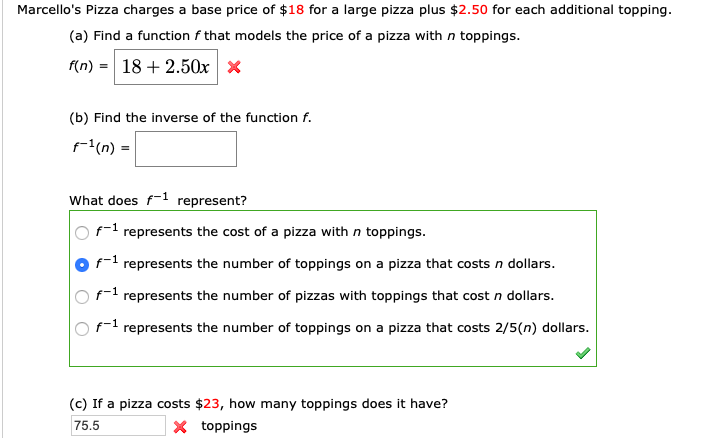# Marcello's Pizza charges a base price of $18 for a large pizza plus$2.50 for each additional topping (a) Find a function f that models the price of a pizza with n toppings. 18 2.50x f(n) (b) Find the inverse of the function f. (n)= What does f represent? f represents the cost of a pizza with n toppings f represents the number of toppings on a pizza that costs n dollars. f1 represents the number of pizzas with toppings that cost n dollars. f represents the number of toppings on a pizza that costs 2/5(n) dollars. (c) If a pizza costs $23, how many toppings does it have? X toppings 75.5 Questionhelp_outlineImage TranscriptioncloseMarcello's Pizza charges a base price of$18 for a large pizza plus $2.50 for each additional topping (a) Find a function f that models the price of a pizza with n toppings. 18 2.50x f(n) (b) Find the inverse of the function f. (n)= What does f represent? f represents the cost of a pizza with n toppings f represents the number of toppings on a pizza that costs n dollars. f1 represents the number of pizzas with toppings that cost n dollars. f represents the number of toppings on a pizza that costs 2/5(n) dollars. (c) If a pizza costs$23, how many toppings does it have? X toppings 75.5 fullscreen

### Want to see this answer and more?

Experts are waiting 24/7 to provide step-by-step solutions in as fast as 30 minutes!*

*Response times vary by subject and question complexity. Median response time is 34 minutes and may be longer for new subjects.
Tagged in
MathCalculus

### Functions